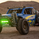8100 vues
Deriving the indicator:

PAI is an indicator I created that tells you the probability of current price moving a specified ATR distance over a specified number of periods into the future. It takes into account 4 variables: the ATR & the standard deviation of price, and the 2 parameters: ATR distance and # bars (time).
The formula is very complex so I will not be able to explain it without confusion arising.

What I can say is that I used integral calculus & the Taylor series to derive a formula that calculates the area under half of the normal distribution function. Thus, the formula was repeated twice in the code to derive the full probability (half + half = whole). If you can read the code, you might be wondering why the formula is so long...

The reason for this is because in Pine Script, the erf function doesn't exist. You see, the formula for normal distribution is: f(x) = (1/sqrt(2pi))*e^(-xx/2), assuming of course that the standard deviation = 1 and mu (mean) = 1. The next step is to take the integral of this formula in order to find the area under f(x). The problem is that I found the integral, F(x), of the normal distribution formula to be equal to F(x) = erf (x/sqrt(2))/2...and the erf function cannot be directly computed into Pinescript.

So I developed a solution...why not estimate the integral function? So that's exactly what I did using a technique involving the Taylor series. The Taylor series is an algebraic function that allows you to create a new function that can estimate the existing function. On a graph, the new function has the same values as the existing one, the only difference is that it uses a differnt formula, in this case, a formula that makes it possible to compute the integral. The disadvantage of using this new formula is that it is super long and if you want it to better represent the original integral over a wider range of x-values, you have to make it longer.

Signal Interpretion:
The hotter the colour, the more likely price will reach your specified distance.
The 2 values of PAI in the bottom window represent probability & average probability of your specifed distance geting hit.

Applications:
Stop loss placement---
This indicator is useful because it gives you an idea of the likelihood that a stop loss at a particular distance away from price (in ATRs ) will be hit over a period of time specified. This is helpful in placing stop losses.
PAI can also be used in options trading. For example, you are using a strangle options strategy, and you want to make sure that price stays within the Strangle's profit range. So you only trade when PAI presents a low probability value of moving at a particular distance in ATRs over n periods.

Anyhow, I hope you guys like it. Enjoy! and hit that like button for me :)
Notes de version: Added limit to 2nd parameter (time).
Notes de version: ...
The indicator now uses a formula that is much simplier and more accurate that the previous formula I derived using the Taylor Series.
It no longer uses the Taylor Series to approximate the erf function: (1/2)*erf(x/sqrt2)
This also means that the indicator will no longer spit out any nan values...so now every probability is known.
Notes de version: Probabilities are now rounded to nearest percent. Histogram is now only 1 color.
Script open-source

Dans le plus pur esprit de TradingView, l'auteur de ce texte l'a publié en open-source, afin que les traders puissent le comprendre et le vérifier. Bravo à l'auteur ! Vous pouvez l'utiliser gratuitement, mais la réutilisation de ce code dans une publication est régie par les règles internes. Vous pouvez le mettre en favori pour l'utiliser sur un graphique.

Vous voulez utiliser ce script sur un graphique ?

## CommentairesPineCoders
@PineCoders, Whoa! What a nice surprise gift. Thank you :D
Répondre"The problem is that I found the integral, F(x), of the normal distribution formula to be equal to F(x) = erf (x/sqrt(2))/2...and the erf function cannot be directly computed into Pinescript." are u sure? Problem for some is opportunity for others i guess. Also, ATR?
Répondrebalipour
Idk man, but the nature of the erf function makes it hard to compute without over complicating things, and I like to keep my code as simple & clean as possible.
ATR...yea I prefer to measure things in ATRs as oppose to absolute prices because it's an objective measure that adapts to price volatility. So for example, a move of 2 ATRs in Gold is the same as a move of 2 ATRs in the EURUSD pair. Hope this helps :D
RépondrePrize_Fighter
@Prize_Fighter, sure, I'll consider. Thank you for those kind words :D
RépondrePrize_Fighter
@Prize_Fighter, good news...it got accepted into editors picks!
Répondre